2021-04-20 14:14:23

全国空气动力测控技术交流会论文集

基于SlMUUNK的FMCW雷达测距功能仿真

也esimulation function

ofFMCW

distancebasedon

ofmeasuring SIMUL腿

徐小平

(中国工程物理研究院电子工程研究所621900)

真模型的建立．对仿真结果进行分析后得出了预期的结论．该算法采用垒敷字化信号处理彤式，性能上满

足测距精度高，稳定性好等技术要求．作为FPGA实现的上层设计，这一方法简单易学、具有很高的实用

价值。

FMCW

thearithmeticofhowtorealize thefunction

abstractThe distance ofFMCWbasedon

paperexplain measuring system

select establish

essentialityofhowto

modelofthe and theresult achievethe

the We result these

system expecting

analyse ofsimulating through

signalprocessinginrespectthatthe

method suchmeritas distanceand ectAsthe

possess high

precisionofmeasuringgoodstability superstratum

methodhasmuchvalueinallkinds

ofFPGA，the

design ofapplication

FMCWMATLAB

signal

key processing

用户提供了一种封装子系统的功能，用户可以自己定义子系统的图标和设置参数对话框；和实际示

波器输出相似的图形化显示结果功能等。对一个简单的设计，就像搭积木块一样方便有趣。对于复

现FMCW雷达的信号处理的具体过程。

1 基本原理

FMCW雷达的测距原理是利用差拍信号实现对高度的测量。其简单原理框图如下：

图I调频冒达

更多相关内容
• 毫米波 （millimeter wave ）波长为1～10毫米的电磁波称毫米波，它位于微波与远红外波相交叠的波长范围，因而兼有两种波谱的特点。　它具有以下主要特点：　?极宽的带宽：通常认为毫米波频率范围为26.5～300GHz，...
• FMCW测距测速使用的matlab代码，希望对大家有帮助
• 在与测距相关的应用中，雷达一般工作在FMCW模式，其原理是将经过调制的连续波信号以较高的载频发射出去，遇到被测目标时，将接收到的回波信号与当下发射的高频信号进行混频得到差频信号。对于线性调频来说，差频的...

在与测距相关的应用中，雷达一般工作在FMCW模式，其原理是将经过调制的连续波信号以较高的载频发射出去，遇到被测目标时，将接收到的回波信号与当下发射的高频信号进行混频得到差频信号。对于线性调频来说，差频的频率即携带着目标的距离信息

## 雷达和测量目标相对静止

当雷达和测量目标相对静止，回波信号和发射信号相比，在时间上延迟了 τ \tau ，可表示为：
τ = 2 R c \tau =\frac{2R}{c}
其中，R为雷达与目标物体的距离，c为光速。
下图为发射信号与回波信号的简化模型图。其中实线部分为发射信号频率曲线 f t f_{t} ,虚线部分为回波信号频率曲线 f r f_{r} ,混频输出的差频信号频率 f b f_{b} 为发射信号和回波信号的频率差.从图中可以得到
tan ⁡ θ = B / 2 T / 4 = f b τ \tan \theta =\frac{B/2}{T/4}=\frac{{{f}_{b}}}{\tau }
可以得到目标物体的距离值R、差频 f b f_{b} 、调频带宽B、调制周期T之间的关系：
R = c T 4 B f b R=\frac{cT}{4B}{{f}_{b}}
当三角波周期T和带宽B为固定的值时，在雷达与目标相对静止时，目标距离与差频 f b f_{b} 成正比。因此，可以通过对差频信号 f b f_{b} 的检测来获取目标距离R。

## 雷达和测量目标之间存在相对运动

多普勒效应指出，波在波源向观察者接近时接收频率变高，而在波源远离观察者时接收频率变低。当观察者移动时也能得到同样的结论。同理，用雷达观测运动目标时也会存在多普勒效应：当目标接近雷达时，雷达的接收频率变高；当目标远离雷达时，雷达的接收频率会变低。
假设雷达和目标接近，在回波信号中包含着由于目标的运动带来的多普勒频移 f d f_{d} 信号。可以得出在三角波的上升沿和下降沿差频信号的频率可分别表示如下：
f b + = f b − f d {{f}_{b+}}={{f}_{b}}-{{f}_{d}}
f b − = f b + f d {{f}_{b-}}={{f}_{b}}+{{f}_{d}}
其中， f b f_{b} 为雷达和目标物体是相对静止时的差频信号的频率，而 f d f_{d} 为目标运动带来的多普勒频移信号。根据多普勒原理，多普勒频移 f d f_{d} 可以表示为
f d = 2 f 0 v c {{f}_{d}}=\frac{2{{f}_{0}}v}{c}
其中， f 0 f_{0} 为发射信号中心频率。上图为雷达与目标存在相对运动时的（a）发射信号与回波信号（b）差频信号
可以得到三角波上升沿所对应的差频 f b + {{f}_{b+}} 和三角波下降沿所对应的差频 f b − {{f}_{b-}}
f b + = 4 B R c T − 2 f 0 v c {{f}_{b+}}=\frac{4BR}{cT}-\frac{2{{f}_{0}}v}{c}
f b − = 4 B R c T + 2 f 0 v c {{f}_{b-}}=\frac{4BR}{cT}+\frac{2{{f}_{0}}v}{c}
则目标距离和速度的表达式为
R = c T 8 B ( f b − + f b + ) R=\frac{cT}{8B}\left( {{f}_{b-}}+{{f}_{b+}} \right)
v = c 4 f 0 ( f b − − f b + ) v=\frac{c}{4{{f}_{0}}}\left( {{f}_{b-}}-{{f}_{b+}} \right)
因此，可以通过 f b + {{f}_{b+}} f b − {{f}_{b-}} 来计算距离 R R 和速度 v v 的值

## 单个静止目标回波信号的时域-频域分析

对于同一时刻，在三角波的上升沿，回波信号的频率低于发射信号的频率。在三角波的下降沿，雷达回波信号的频率则会高于发射信号频率。所以，单个目标的差频信号会围绕着三角波的峰顶和峰谷处呈现对称形式。整个差频信号则是一个周期性信号，周期等于三角波的调制周期T。
x ( t ) x\left( t \right) 为差频时域信号， s ( t ) s\left( t \right) 为三角波上升沿所对应的差频时域信号，则三角波下降沿所对应的差频时域信号为 s ( T − t ) s\left( T-t \right) 。对差频信号进行时域数学建模，即
x ( t ) = { s ( t ) ,             t ∈ [ 0 , T 2 ] + n T , n = 0 , 1 , 2... s ( T − t )   ,    t   ∈ [ T 2 , T ]   + n T , n = 0 , 1 , 2... x\left( t \right)=\left\{ \begin{aligned} & s\left( t \right),\ \ \ \ \ \ \ \ \ \ \ t\in \left[ 0,\frac{T}{2} \right]+nT,n=0,1,2... \\ & s\left( T-t \right)\ ,\ \ t\ \in \left[ \frac{T}{2},T \right]\ +nT,n=0,1,2... \\ \end{aligned} \right.
由于差频信号是周期信号，周期信号可以展开成傅里叶级数的形式
x ( t ) = ∑ k = - ∞ ∞ A k e j k Ω T t x\left( t \right)=\sum\limits_{k=\text{-}\infty }^{\infty }{{{A}_{k}}}{{e}^{jk{{\Omega }_{T}}t}}
其中， Ω T = 2 π T {{\Omega }_{T}}=\frac{2\pi }{T} A k {{A}_{k}} 为傅里叶级数的系数。
系数 A k {{A}_{k}} 可以通过如下公式来求解
A k = 1 T ∫ 0 T x ( t ) e − j k Ω T t d t = 1 T ∫ 0 T 2 s ( t ) e − j k Ω T t d t + 1 T ∫ T 2 T s ( T − t ) e − j k Ω T t d t {{A}_{k}}=\frac{1}{T}\int_{0}^{T}{x\left( t \right)}{{e}^{-jk{{\Omega }_{T}}t}}dt=\frac{1}{T}\int_{0}^{\frac{T}{2}}{s\left( t \right)}{{e}^{-jk{{\Omega }_{T}}t}}dt+\frac{1}{T}\int_{\frac{T}{2}}^{T}{s\left( T-t \right)}{{e}^{-jk{{\Omega }_{T}}t}}dt
对上式的第二项进行变量替换
1 T ∫ T 2 T s ( T − t ) e − j k Ω T t d t = t ′ = T − t   − 1 T e − j k Ω T T ∫ T 2 0 s ( t ′ ) e j k Ω T t ′ d t ′ = t = t ′   − 1 T ∫ T 2 0 s ( t ) e j k Ω T t d t = 1 T ∫ 0 T 2 s ( t ) e j k Ω T t d t \begin{aligned} & \frac{1}{T}\int_{\frac{T}{2}}^{T}{s\left( T-t \right)}{{e}^{-jk{{\Omega }_{T}}t}}dt\overset{t'=T-t}{\mathop{=}}\,-\frac{1}{T}{{e}^{-jk{{\Omega }_{T}}T}}\int_{\frac{T}{2}}^{0}{s\left( t' \right)}{{e}^{jk{{\Omega }_{T}}t'}}dt' \\ & \overset{t=t'}{\mathop{=}}\,-\frac{1}{T}\int_{\frac{T}{2}}^{0}{s\left( t \right)}{{e}^{jk{{\Omega }_{T}}t}}dt=\frac{1}{T}\int_{0}^{\frac{T}{2}}{s\left( t \right)}{{e}^{jk{{\Omega }_{T}}t}}dt \\ \end{aligned}

A k = 1 T ∫ 0 T 2 s ( t ) e − j k Ω T t d t + 1 T ∫ 0 T 2 s ( t ) e j k Ω T t d t = 1 T ∫ 0 T 2 s ( t ) e − j k Ω T t d t + 1 T ∫ 0 T 2 s ( t ) e − j ( − k Ω T ) t d t \begin{aligned} & {{A}_{k}}=\frac{1}{T}\int_{0}^{\frac{T}{2}}{s\left( t \right)}{{e}^{-jk{{\Omega }_{T}}t}}dt+\frac{1}{T}\int_{0}^{\frac{T}{2}}{s\left( t \right)}{{e}^{jk{{\Omega }_{T}}t}}dt \\ & =\frac{1}{T}\int_{0}^{\frac{T}{2}}{s\left( t \right)}{{e}^{-jk{{\Omega }_{T}}t}}dt+\frac{1}{T}\int_{0}^{\frac{T}{2}}{s\left( t \right)}{{e}^{-j\left( -k{{\Omega }_{T}} \right)t}}dt \\ \end{aligned}
其中，第一项是信号 s ( t ) s\left( t \right) 被时间窗口函数 R T / 2 = { 1      t ∈ [ 0 , T 2 ] 0      t ∈ o t h e r {{R}_{T/2}}=\left\{ \begin{aligned} & 1\ \ \ \ t\in \left[ 0,\frac{T}{2} \right] \\ & 0\ \ \ \ t\in other\\ \end{aligned} \right. ，然后信号做傅里叶变换在 Ω = k Ω T \Omega =k{{\Omega }_{T}} 处取值的结果。第二项则是在 Ω = − k Ω T \Omega =-k{{\Omega }_{T}} 处取值的结果。
上式可以进一步表示为
A k = 1 T ∫ 0 T 2 s ( t ) e − j k Ω T t d t + 1 T ∫ 0 T 2 s ( t ) e j k Ω T t d t = 2 T ∫ 0 T 2 s ( t ) cos ⁡ ( k Ω T t ) d t \begin{aligned} & {{A}_{k}}=\frac{1}{T}\int_{0}^{\frac{T}{2}}{s\left( t \right)}{{e}^{-jk{{\Omega }_{T}}t}}dt+\frac{1}{T}\int_{0}^{\frac{T}{2}}{s\left( t \right)}{{e}^{jk{{\Omega }_{T}}t}}dt \\ & =\frac{2}{T}\int_{0}^{\frac{T}{2}}{s\left( t \right)}\cos \left( k{{\Omega }_{T}}t \right)dt \\ \end{aligned}
对于单个检测目标，设差频信号为单一频率的信号，则上升沿所对应的差频时域信号可表示为：
s ( t ) = cos ⁡ ( 2 π f b t + φ ) = cos ⁡ ( Ω b t + φ ) s\left( t \right)=\cos \left( 2\pi {{f}_{b}}t+\varphi \right)=\cos \left( {{\Omega }_{b}}t+\varphi \right)
其中， φ \varphi 是相位， Ω b {{\Omega }_{b}} 为差频信号 f b f_{b} 所对应的模拟角频率。那么
A k = 2 T ∫ 0 T 2 cos ⁡ ( k Ω T t ) cos ⁡ ( Ω b t + φ ) d t = 1 T ∫ 0 T 2 cos ⁡ [ ( k Ω T + Ω b ) t + φ ] d t + 1 T ∫ 0 T 2 cos ⁡ [ ( k Ω T − Ω b ) t − φ ] d t = 1 T ( k Ω T + Ω b ) [ sin ⁡ [ ( k Ω T + Ω b ) T 2 + φ ] − sin ⁡ φ ] + 1 T ( k Ω T − Ω b ) [ sin ⁡ [ ( k Ω T − Ω b ) T 2 − φ ] + sin ⁡ φ ] \begin{aligned} & {{A}_{k}}=\frac{2}{T}\int_{0}^{\frac{T}{2}}{\cos \left( k{{\Omega }_{T}}t \right)\cos \left( {{\Omega }_{b}}t+\varphi \right)}dt \\ & =\frac{1}{T}\int_{0}^{\frac{T}{2}}{\cos \left[ \left( k{{\Omega }_{T}}+{{\Omega }_{b}} \right)t+\varphi \right]}dt+\frac{1}{T}\int_{0}^{\frac{T}{2}}{\cos \left[ \left( k{{\Omega }_{T}}-{{\Omega }_{b}} \right)t-\varphi \right]}dt \\ & \text{=}\frac{1}{T\left( k{{\Omega }_{T}}+{{\Omega }_{b}} \right)}\left[ \sin \left[ \left( k{{\Omega }_{T}}+{{\Omega }_{b}} \right)\frac{T}{2}+\varphi \right]-\sin \varphi \right] \\ & +\frac{1}{T\left( k{{\Omega }_{T}}-{{\Omega }_{b}} \right)}\left[ \sin \left[ \left( k{{\Omega }_{T}}-{{\Omega }_{b}} \right)\frac{T}{2}-\varphi \right]+\sin \varphi \right] \\ \end{aligned}
不妨设 φ = 0 \varphi \text{=}0 ，则
A k = 1 2 sin ⁡ [ ( k Ω T + Ω b ) T 2 ] ( k Ω T + Ω b ) T 2 + 1 2 sin ⁡ [ ( k Ω T − Ω b ) T 2 ] ( k Ω T − Ω b ) T 2 = 1 2 sin ⁡ [ ( Ω + Ω b ) T 2 ] ( Ω + Ω b ) T 2 ∣ Ω = k Ω T + 1 2 sin ⁡ [ ( Ω − Ω b ) T 2 ] ( Ω − Ω b ) T 2 ∣ Ω = k Ω T \begin{aligned} & {{A}_{k}}=\frac{1}{2}\frac{\sin \left[ \left( k{{\Omega }_{T}}+{{\Omega }_{b}} \right)\frac{T}{2} \right]}{\left( k{{\Omega }_{T}}+{{\Omega }_{b}} \right)\frac{T}{2}}+\frac{1}{2}\frac{\sin \left[ \left( k{{\Omega }_{T}}-{{\Omega }_{b}} \right)\frac{T}{2} \right]}{\left( k{{\Omega }_{T}}-{{\Omega }_{b}} \right)\frac{T}{2}} \\ & ={{\left. \frac{1}{2}\frac{\sin \left[ \left( \Omega +{{\Omega }_{b}} \right)\frac{T}{2} \right]}{\left( \Omega +{{\Omega }_{b}} \right)\frac{T}{2}} \right|}_{\Omega =k{{\Omega }_{T}}}}+{{\left. \frac{1}{2}\frac{\sin \left[ \left( \Omega -{{\Omega }_{b}} \right)\frac{T}{2} \right]}{\left( \Omega -{{\Omega }_{b}} \right)\frac{T}{2}} \right|}_{\Omega =k{{\Omega }_{T}}}} \\ \end{aligned}
可以看出，系数 A k {{A}_{k}} 呈现出辛格函数的包络形式。当 ( k Ω T − Ω b ) ⋅ T / 2 = m π , m = 0 , 1 , 2... \left( k{{\Omega }_{T}}-{{\Omega }_{b}} \right)\cdot T/2=m\pi ,m=0,1,2... 时，或者 ( k Ω T + Ω b ) ⋅ T / 2 = m π , m = 0 , 1 , 2... \left( k{{\Omega }_{T}}+{{\Omega }_{b}} \right)\cdot T/2=m\pi ,m=0,1,2... 时， A k = 0 {{A}_{k}}=0
可以解得 Ω b = ( k − m ) Ω T {{\Omega }_{b}}=\left( k-m \right){{\Omega }_{T}} Ω b = ( m − k ) Ω T {{\Omega }_{b}}=\left( m-k \right){{\Omega }_{T}} 时， A k = 0 {{A}_{k}}=0 。当 Ω b > > Ω T {{\Omega }_{b}}>>{{\Omega }_{T}} 时候， A k {{A}_{k}} 的数值大小的分布如下图所示Ω b = k ⋅ Ω T {{\Omega }_{b}}=k\cdot {{\Omega }_{T}} 时， A k {{A}_{k}} 的大小分布如图中的黑色实线所示， Ω b {{\Omega }_{b}} 恰好落在离散的整数频点上，其所对应的 A k {{A}_{k}} 为最大值。如果 Ω b {{\Omega }_{b}} 落在两个离散的整数频点中间，如图中的红色虚线所示，相当于辛格函数的峰值落在了两个离散整数频点中间，且距离信号实际频率越近的频点 A k {{A}_{k}} 越大。
可以看出 A k {{A}_{k}} 的值的分布随着 Ω b {{\Omega }_{b}} 的变化而变化，且包络呈现辛格函数的形状。因此，FMCW体制雷达的差频信号的傅里叶变换是一个傅里叶级数的形式，频谱上呈现离散状态，且可以通过找寻 A k {{A}_{k}} 的最大值来确定或者近似确定 Ω b {{\Omega }_{b}} 的值。

## 雷达距离分辨率

当两个目标位于同一方位角，但与雷达的距离不同时，二者被雷达区分出来的最小距离称为距离分辨率。通常定义为：当较近目标回波脉冲的后沿（下降沿）与较远目标回波的前沿（上升沿）刚好重合时，作为可分辨的极限。此时两目标间的距离就是距离分辨率，常用 △ R \vartriangle R 表示
Δ R = c T 4 B Δ f b \Delta R=\frac{cT}{4B}\Delta {{f}_{b}}
其中， Δ f b \Delta {{f}_{b}} 为差频分辨率。一般情况下，差频信号的周期应该小于三角波周期的一半，即
f b ≥ 2 T {{f}_{b}}\ge \frac{2}{T}
那么
Δ R ≥ c T 4 B ⋅ 2 T = c 2 B \Delta R\ge \frac{cT}{4B}\cdot \frac{2}{T}=\frac{c}{2B}
距离分辨率和系统的带宽B有关

## 雷达差频信号仿真

给出雷达差频信号的仿真，参数设置如下：

参数名称参数值
三角板周期1KHz
采样频率1MHz
中心频率20KHz
调制带宽2KHz

可以得到三角波信号如下：调制后的信号（发射信号为）：回波信号为：那么相应的差频信号，即实际低通滤波后的信号，可以表示为：从仿真的结果也可以看出与上面的分析一致。

展开全文• ## FMCW雷达原理

千次阅读 2021-05-24 18:03:28
FMCW雷达系统通过天线向外发射一列连续调频毫米波，并接收目标的反射信号。发射波的频率在时域中按调制电压的规律变化。FMCW毫米波雷达的发射信号采用的是频率调制，常用的调制信号有：正弦波信号、锯齿波信号和三角...

FMCW即Frequency Modulated Continuous Wave，译为调频连续波。FMCW 雷达系统通过天线向外发射一列连续调频毫米波，并接收目标的反射信号。发射波的频率在时域中按调制电压的规律变化。FMCW 毫米波雷达的发射信号采用的是频率调制，常用的调制信号有：正弦波信号、锯齿波信号和三角波信号等，当以三角波或锯齿波作为调频波时，称其为线性调频连续波（LFMCW）。三角波线性调频连续波利用差拍傅立叶方式在一个周期内就可无模糊确定目标距离和速度，处理简单，易于实现，它利用发射信号的线性调频和从目标反射回来的接收信号频率的变化相关和频谱配对来进行动目标的测量，比较易于实现的测距测速连续波雷达，因此三角波线性调频连续波雷达的设计和实现，有着非常重要的现实意义。

# FMCW雷达框图

调频连续波雷达如要由收发器和带微处理器的控制单元组成，收发器如果使用单个天线进行同时发射和接收，FMCW雷达需要铁氧体环形器来分离发射和接收信号，对隔离度要求较高。当然，若使用收发分离的贴片天线，成本会相对低一点。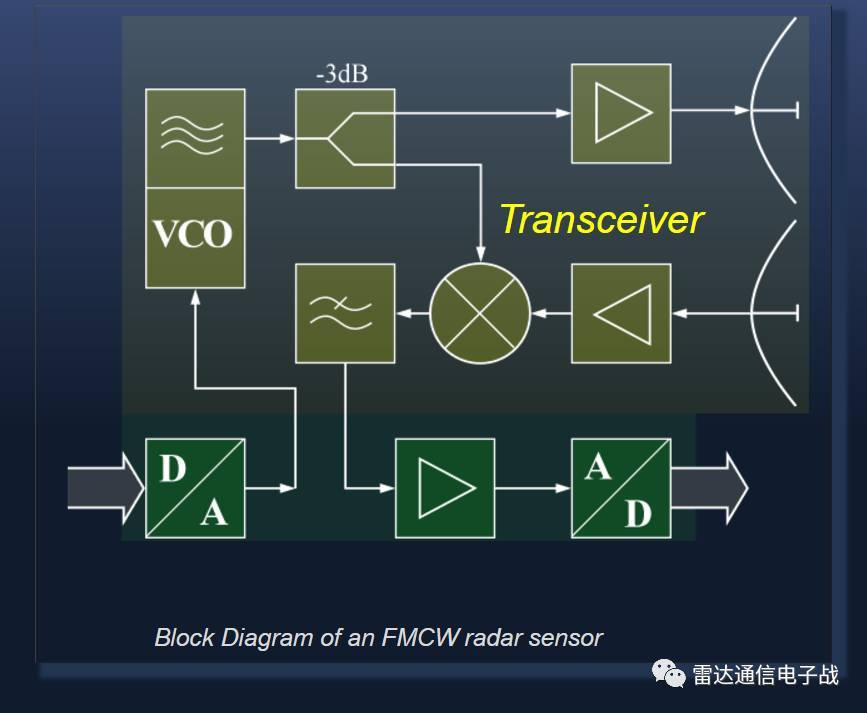高频信号由压控振荡器(VCO)产生，通过功率分配器将一部分经过额外放大后馈送至发射天线，另一部分耦合至混频器，与接收的回波混频、低通滤波，得到基带差频信号，经过模数转换后送至微处理器处理。

# FMCW雷达的测距/测速原理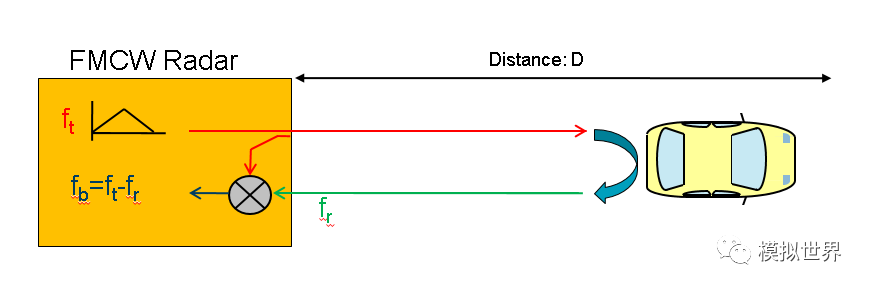LFMCW 波雷达的工作原理是用回波信号和发射信号的一部分进行相干混频，得到包含目标的距离和速度信息的中频信号，然后对中频信号进行检测即可得到目标的距离和速度。当目标物体是相对静止的，发射信号碰到目标物体后被反射回来，产生回波信号，回波信号与发射信号形状相同，只是在时间上延迟了τ（τ=2R/c），式中：R-目标物体的距离；c-光速。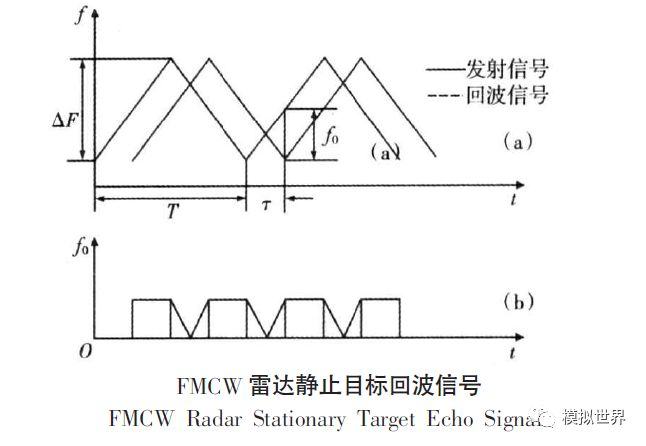发射信号与回波信号的频率差即为混频输出的中频信号频率f0，根据相似三角形的关系，由上图（a）可以得出：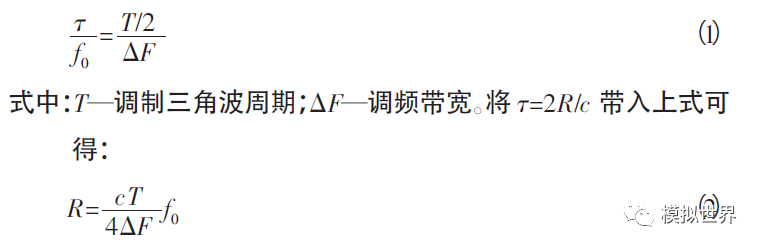从上式中可以看出，在调制周期T 和调频带宽确定的情况下，目标距离与LFMCW 雷达前端混频器输出的中频信号频率成正比，这就是目标物体处于相对静止的情况下LFMCW 雷达测距原理。

从上式中可以看出，在调制周期T 和调频带宽确定的情况下，目标距离与LFMCW 雷达前端混频器输出的中频信号频率成正比，这就是目标物体处于相对静止的情况下LFMCW 雷达测距原理。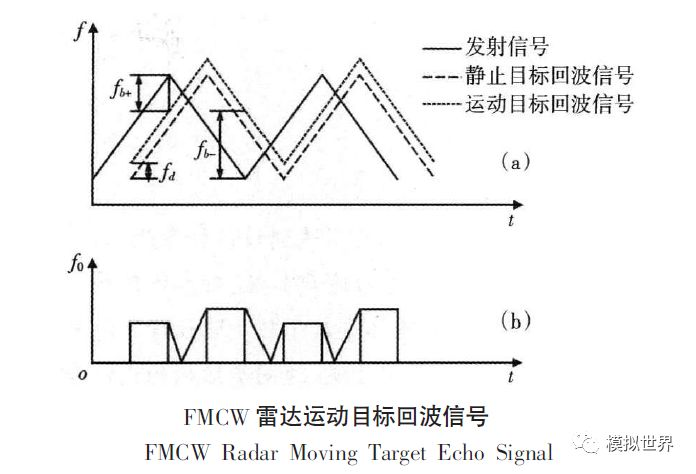从上图中可以看出，三角波的上升沿和下降沿中频信号的频率可分别表示如下：

fb+=f0-fd

fb-=f0+fd

上两式中为目标物体相对静止时中频信号的频率，为多普勒频移。由距离公式R和多普勒频移公式可得：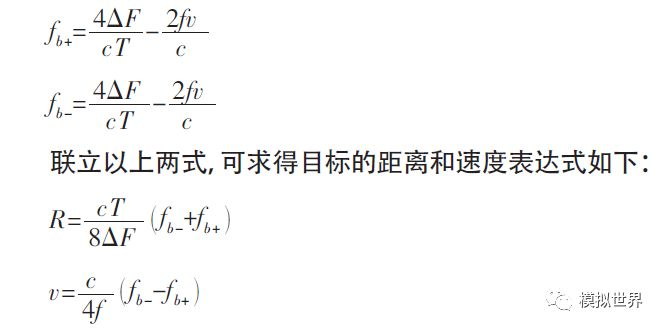由上述公式可以看出，毫米波雷达信号中频频率fb的确定是求出R、V的关键。

以上两式虽然是在目标处于相对运动状态下推导出来的，但是对于相对静止的目标同样适用，因此，在实际应用中，不管目标是处于相对运动还是相对静止，只要分别求出调制三角波在上升沿和下降沿的中频信号的频率，就可以利用以上两式来计算目标的距离和速度信息，这就是FMCW 毫米波雷达测距测速原理。

f的确定即是对发射和反射信号的频差进行频谱分析。信号的频谱分析主要有FFT法和非FFT法。所谓FFT法，即是对被分析的信号进行傅里叶变换，将其从时域变到频域，在频域进行分析，必要时再通过傅里叶逆变换，变回时域的分析方法。而非FFT方法则是通过其他的途径，获得信号的频率参数，如最大熵法、MUSIC法等，各有特点。综合考虑方法的复杂性，实时性，稳定性，对汽车雷达而言，频谱分析应首选FFT法，这种方法比较成熟，实现容易，实时性强，适合于汽车运行状况下信号的实时处理。

当雷达的辐射范围内有多个目标时，三角波调频方式雷达会产生一定的虚警率。

当前方为单目标时，由前面的公式，可以确定两个二元一次方程。在R—V平面内会确定两条斜率相反的直线，如下图中两条实线。从而获得一个交点，如下图中实线的交点，即可确定目标的相对速度和距离信息。而在多目标监测时则会产生虚警。以两个目标为例，由于雷达的参数相同(k。，k：相同)，两个目标在发射信号处于频率上升和下降的频段会产生两组平行的直线，有四个交点，其中两个为虚警目标；如下图所指出，根据中频信号的信息无法排除。随着目标数量的增加，虚假目标会成倍的增长。因此，必须对雷达的发射波形进行调整，调整一方面应提高雷达的多目标分辨率，一方面要算法简便快捷，保证系统的实时性。波形调整的方法有很多种，至今也是毫米波汽车雷达应用的一个难点。这里仅对增加频率段方法的原理做一简单阐述。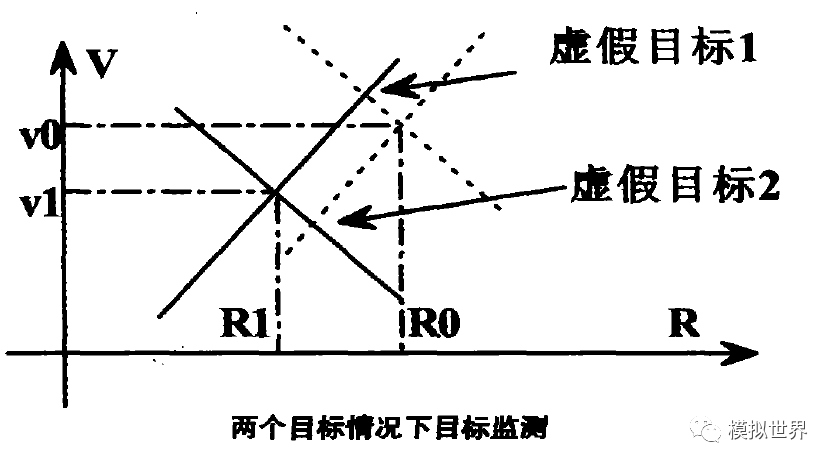由于增加了和原有频率不同斜率的发射信号，如下图中的C段，则目标点在R--V平面内将获得另外一条直线，与原来两条直线交于一点，即为目标的信息点，如下图两目标检测中实线的交点。当遇到多目标时如果分属于不同的频率段的直线交于一点，则该点为真实目标点。以两目标点为例，如下图所示，三直线相交与一点，即为真实目标点，从而消除了一定的虚假目标。当然，增加的频率段越多，则可以消除的虚假目标也就越多。但是，每增加一个频率段就会增加系统的运算时间，为此频率段的增加和系统的实时性要有一定的折中。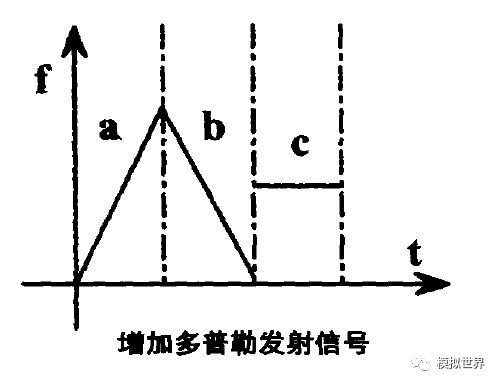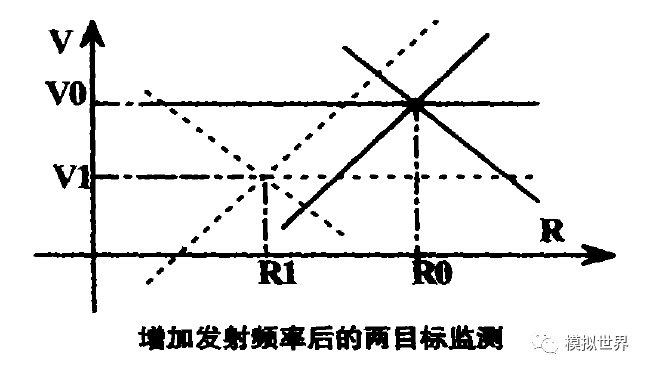随着CMOS高频器件和单片微波集成电路 MMIC的出现和应用，毫米波雷达的性能有了很大的提高，成本也有所下降，并且雷达的外型尺寸可以做得很小，便于在汽车上安装。因此，毫米波雷达就成了汽车前视雷达的首选。为了在高速公路上及时发现前方的交通堵塞，汽车用毫米波雷达的探测距离必须在100m以上；为了覆盖左右两侧的车道线，探测宽度必为3.5m；为了不把道路上方的标识和人行天桥也探测进去，上、下方要有与道路的升降相对应的3m左右的探测幅度。

# 参考文献

展开全文算法
• 基于FMCW的超声实时测距技术，后续将分享matlab代码。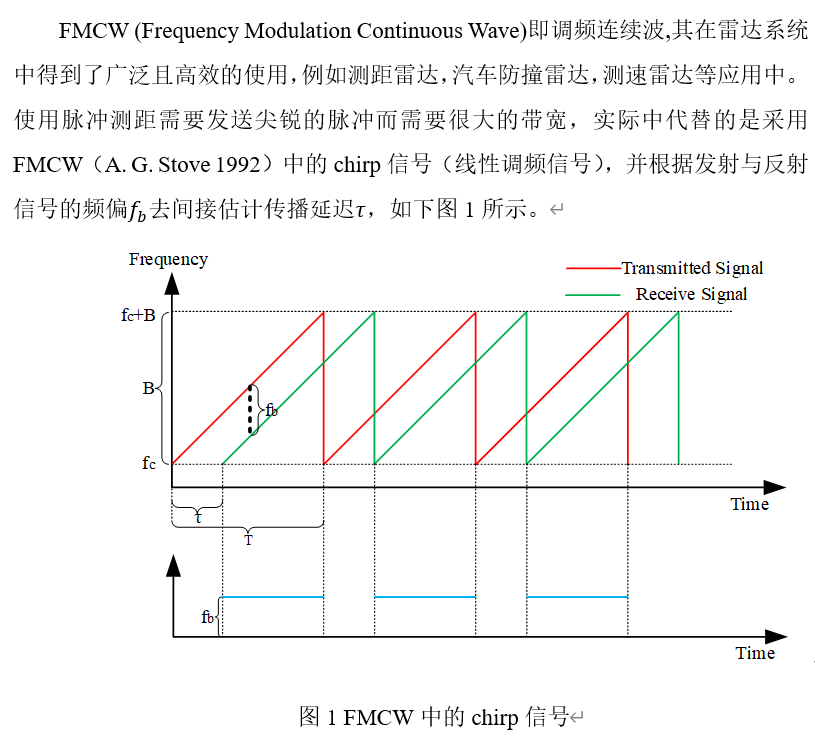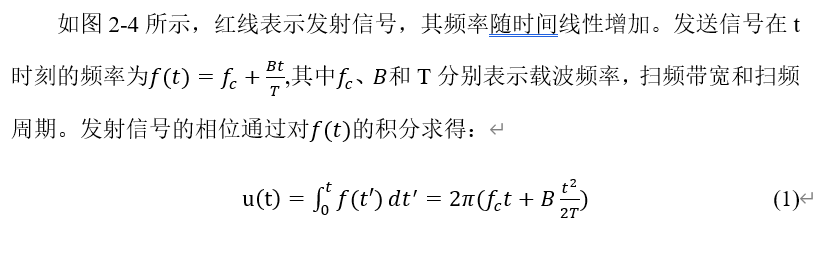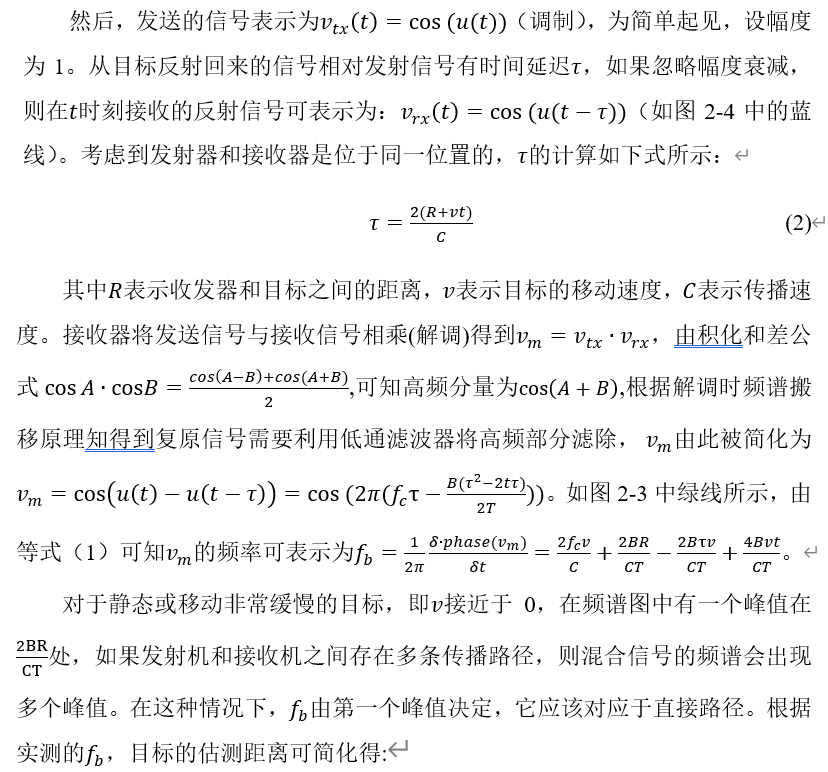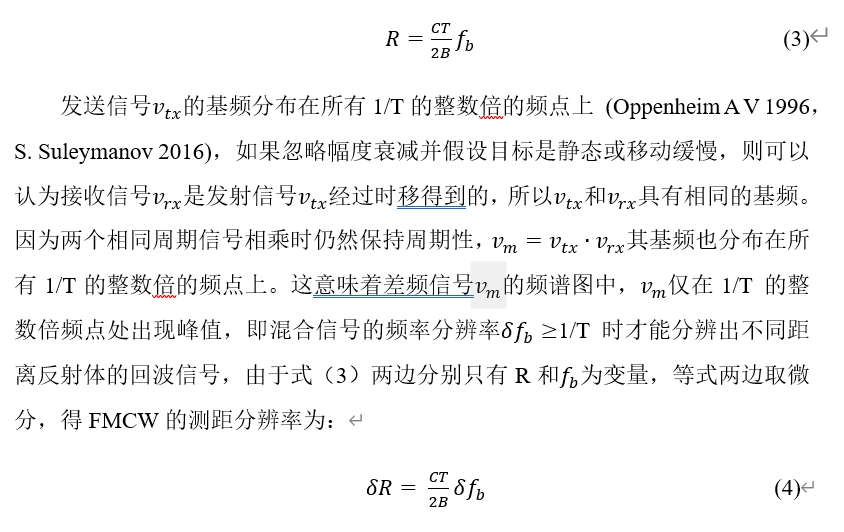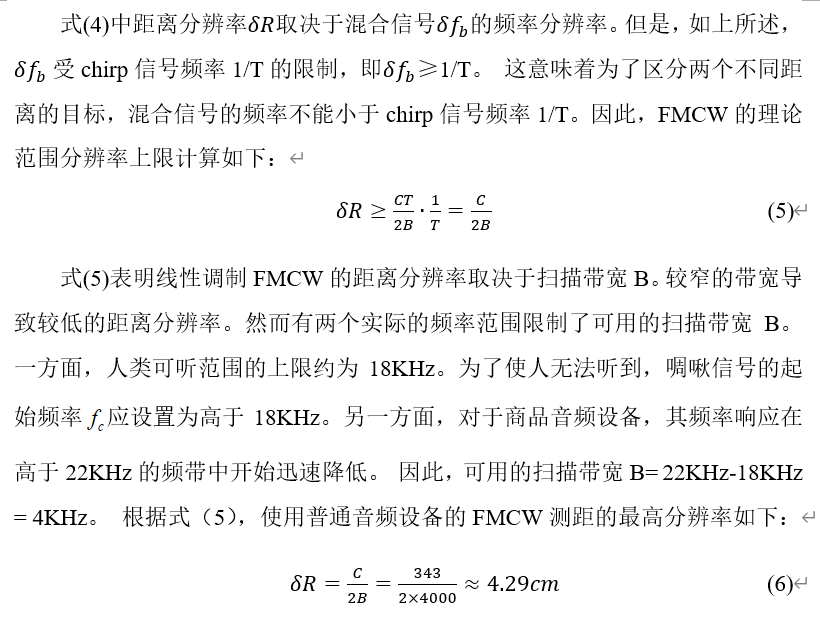展开全文matlab
• ## FMCW雷达基本原理

万次阅读 2018-10-18 16:23:24
最近Infineon和TI都出了FMCW模块，雷达的应用场合已经不仅仅是军用、汽车了，还包括了飞行器、两轮平衡车。最近推出的20米探测距离以内的24G雷达模块，与LIDAR竞争短量程市场。博主了解到北醒的TFMINI这款LIDAR，200...
• 雷达按照发射信号种类分成脉冲雷达和连续波雷达两大类，常规脉冲雷达...其中，单频连续波雷达仅可用于测速，无法测距，而FMCW雷达既可测距又可测速，并且在近距离测量上的优势日益明显。 FMCW雷达在扫频周期内...
•研究论文
• 提高FMCW雷达测距精度的谱最大值估值算法整理.pdf
• FMCW实现测距 系统框图 波形示意 计算公式 则 代入 得 由上，测距实际上是通过发射信号与回波信号的差频计算出距离。差频的值可以通过对差频信号做傅里叶变换得到。 matlab仿真 发射信号与回波信号 t1=0:pi/...matlab 算法 傅立叶分析
• FMCW技术和脉冲雷达技术是两种在高精度雷达测距中使用的技术。其基本原理为，发射波为高频连续波，其频率随时间按照三角波规律变化。雷达接收的回波的频率与发射的频率变化规律相同，都是三角波规律，只是有一个时间...
• 1 FMCW雷达基本框架 2FMCW原理介绍 1 FMCW雷达基本框架 调频连续波雷达的基本框图如图 1所示，框架中主要包括上位机显示与控制界面、信号处理机、收发支路以及天线四个部分。 1)上位机显示与控制界面...
• 1 毫米波雷达测距原理 （1）FMCW雷达的核心：chirp 频率fC开始的线性调频脉冲，4GHZ的带宽，最终以81GHZ的频率结束。 线性调频脉冲的斜率S：线性调频脉冲上升的速率。 带宽B和 斜率S是系统性能的重要参数。 ...
• 为了研制汽车防撞系统，设计了一款24 GHz FMCW雷达系统。该系统包括雷达射频模块、中频信号处理模块和数字基带处理模块。雷达射频模块采用基于异质结场效应晶体管NE3514研制的雷达射频模块传感器实现；中频信号处理...
• 24GHz FMCW车载测距雷达系统设计.pdf，介绍FMCW原理，设计等。
• ## FMCW毫米波雷达原理

千次阅读 2021-04-11 07:51:20
雷达测速原理 雷达主要利用多普勒频移来测量移动物体的速度，即雷达向前发射毫米波波段的电磁波，并接收其回波；如遇到移动物体，则回波频率与发射频率会出现偏差，即多普勒频移；利用此频移即可获得相对速度。...
• 77GHz调频连续波雷达，matlab仿真。该仿真模拟了雷达信号传输过程和回波信号处理过程。
• 本章摘要：介绍什么是调频连续波（FMCW），它是如何进行测距的，测距分辨率分析，测距范围分析。
• 算法主要用于雷达测距，含FFT 和 CZT算法，联合估计频率值
• ## 锯齿波FMCW雷达目标检测原理

万次阅读 多人点赞 2019-06-18 23:03:45
FMCW（Frequency Modulated Continuous Wave），即调频的连续波信号。目前在安防、自动驾驶等领域的应用极为广泛。本文从最基本的数学原理出发进行推导，对锯齿波体制FMCW雷达目标检测进行详细说明。目标检测
• 利用MATLAB仿真FMCW雷达测距测角的过程，对学习FMCW雷达有一定的帮助，能够进一步了解该过程
• FMCW技术和脉冲雷达技术是两种在高精度雷达测距中使用的技术。其基本原理为发射波为高频连续波，其频率随时间按照三角波规律变化。 1.基础知识 FMCW雷达的核心是一种叫做线性调频脉冲的信号,线性调频脉冲是频率随...物联网
• 针对调频连续波（FMCW雷达回波信号中存在噪声的问题，采用小波阈值法进行去噪。详细讨论了小波阈值去噪过程中各参数的选取原则，构造了一种优于典型阈值函数（如：软、硬阈值函数，软硬阈值改良折衷法和半软阈值...
• 这是一篇简单介绍基于单边带光调制器的调频连续波激光测距整理。笔记首先回顾了并行马赫-曾德干涉 (MachZender inteference， MZI) 单边带调制器的工作原理，以及光电平衡探测器的工作特点，最后介绍了包含调频连续...自动驾驶 激光雷达
• 雷达原理笔记之LFMCW雷达测距测速 ——南京理工大学许志勇老师的《雷达原理课程》浅析 文章目录雷达原理笔记之LFMCW雷达测距测速@[toc]1.单边扫频锯齿波1.1静止目标回波分析1.2运动目标回波分析1.3优缺点分析2.双边......